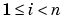MATLAB Function Referencesize

Array dimensions

Syntax

• ```d = size(X)
[m,n] = size(X)
m = size(X`,`dim)
[d1,d2,d3,...,dn] = size(X)
```

Description

```d = size(X) ``` returns the sizes of each dimension of array `X` in a vector `d` with `ndims(X)` elements. If `X` is a scalar, which MATLAB regards as a 1-by-1 array, `size(X)` returns the vector `[1 1]`.

```[m,n] = size(X) ``` returns the size of matrix `X` in separate variables `m` and `n`.

```m = size(X,dim) ``` returns the size of the dimension of `X` specified by scalar `dim`.

```[d1,d2,d3,...,dn] = size(X), ``` for `n` > 1, returns the sizes of the dimensions of the array `X` in the variables d1,d2,d3,...,dn, provided the number of output arguments `n` equals `ndims(X)`. If `n` does not equal `ndims(X)`, the following exceptions hold:

 `n < ndims(X)` `di` equals the size of the `i`th dimension of `X` for, but `dn` equals the product of the sizes of the remaining dimensions of `X,`that is, dimensions `n` through `ndims(X)`. `n > ndims(X)` `size` returns ones in the "extra" variables, that is, those corresponding to `ndims(X)+1` through `n`.

 Note    For a Java array, `size` returns the length of the Java array as the number of rows. The number of columns is always 1. For a Java array of arrays, the result describes only the top level array.

Examples

Example 1. The size of the second dimension of `rand(2,3,4)` is 3.

• ```m = size(rand(2,3,4),2)

m =
3
```

Here the size is output as a single vector.

• ```d = size(rand(2,3,4))

d =
2     3     4
```

Here the size of each dimension is assigned to a separate variable.

• ```[m,n,p] = size(rand(2,3,4))
m =
2

n =
3

p =
4
```

Example 2. If X = ones(3,4,5), then

• ```[d1,d2,d3] = size(X)

d1 =       d2 =       d3 =
3          4          5
```

But when the number of output variables is less than ndims(X):

• ```[d1,d2] = size(X)

d1 =       d2 =
3          20
```

The "extra" dimensions are collapsed into a single product.

If n > ndims(X), the "extra" variables all represent singleton dimensions:

• ```[d1,d2,d3,d4,d5,d6] = size(X)

d1 =       d2 =       d3 =
3          4          5

d4 =       d5 =       d6 =
1          1          1
```

`exist`, `length`, `numel`, `whos`